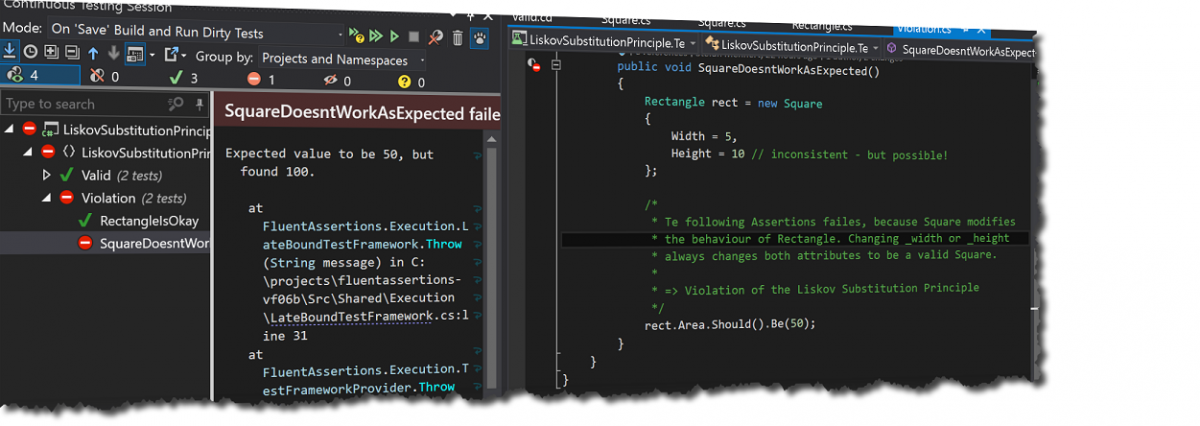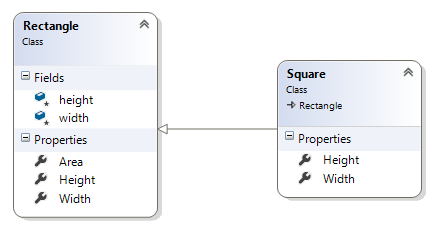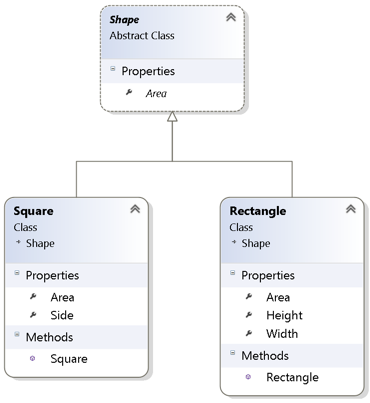Liskov Substitution PrincipleThe Liskov Substitution Principle (LSP) says: “Let$\phi(x)$ be a property provable about objects x of type T. Then$\phi(y)$ should be true for objects y of type S where S is a subtype of T.” (Wikipedia)

That means: A derived class should by able to be replaced by another object of it’s base class without errors or modified behavior of the base class.

What’s the problem?

We tend to model the real world into classes. This is in general a good practice, but it has pitfalls.

Let’s look at an example: A square is an rectangle, so we model it as a subclass of rectangle, so we can handle a square like a rectangle and reuse it’s area property.Ok, let’s have a look at the test’s:

Because we reuse the rectangle class for the square, it’s possible to set width and height separately. Square redefines the width and height setters to set both properties when one of them is changed. In the failing test we create a square with 5×5 by setting the width, and by setting the height we change it to 10×10. This is definitively a violation of the LSP.

How to make it lsp conform

As we’ve learned, rectangle and square are somehow related, but their (implementation) details are different. To encapsulate what varies and to provide a generic interface we introduce an abstract Shape class.

Both implementations have immutable properties and implement the abstract “Area” property get accessor. Because a square is only initialised with the side length, Area works always as expected.
This way we also could add additional shapes like a circle, which would be instantiated with a radius.You find the full example project in my GitHub repository.

Conclusion

Inheritance can lead to very complex and hard to maintain code. Today we use inheritance rarely (Favour composition over inheritance), but when – we should have the LSP in mind.